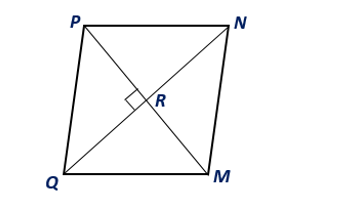Chapter 8.CT, Problem 6CT### Elementary Geometry for College St...

6th Edition
Daniel C. Alexander + 1 other
ISBN: 9781285195698

#### Solutions

Chapter
Section### Elementary Geometry for College St...

6th Edition
Daniel C. Alexander + 1 other
ISBN: 9781285195698
Textbook Problem
1 views

# Find the area of rhombus MNPQ given that Q N = 8 ft and P M = 6 ft.                                   _To determine

To find:

The area of the rhombus MNPQ.

Explanation

Given:

Rhombus MNPQ given that QN=8 ft and PM=6 ft.

Corollary:

The area A of a rhombus whose diagonals have lengths d1 and d2 is given by A=12d1d2.

Calculation:

From the given, let d1=QN=8 ft., and d2=PM=6 ft

### Still sussing out bartleby?

Check out a sample textbook solution.

See a sample solution

#### The Solution to Your Study Problems

Bartleby provides explanations to thousands of textbook problems written by our experts, many with advanced degrees!

Get Started

#### Evaluate 1m when m=67.

Calculus: An Applied Approach (MindTap Course List)

#### Expand each expression in Exercises 122. (x32x2+4)(3x2x+2)

Finite Mathematics and Applied Calculus (MindTap Course List)

#### True or False:

Study Guide for Stewart's Multivariable Calculus, 8th